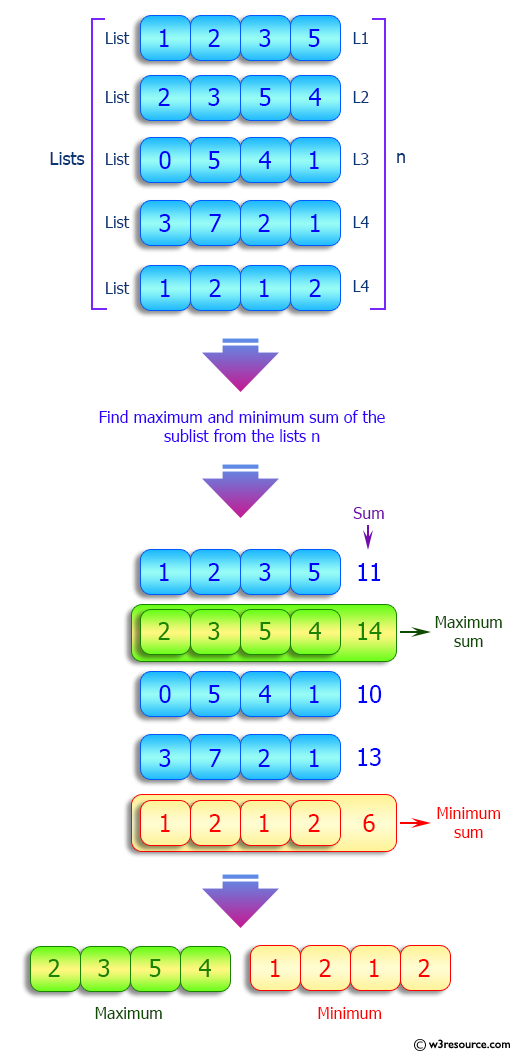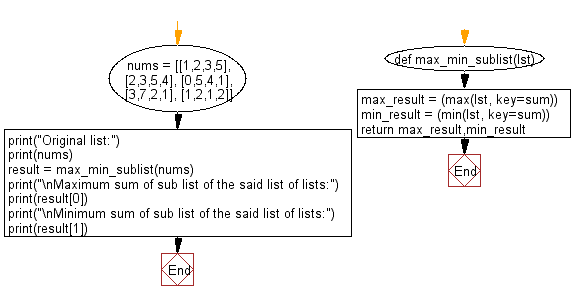﻿ Python: Calculate the maximum and minimum sum of a sublist in a given list of lists - w3resource# Python: Calculate the maximum and minimum sum of a sublist in a given list of lists

## Python List: Exercise - 182 with Solution

Write a Python program to calculate the maximum and minimum sum of a sublist in a given list of lists.

Sample Solution:

Python Code:

``````def max_min_sublist(lst):
max_result = (max(lst, key=sum))
min_result = (min(lst, key=sum))
return max_result,min_result

nums = [[1,2,3,5], [2,3,5,4], [0,5,4,1], [3,7,2,1], [1,2,1,2]]
print("Original list:")
print(nums)
result = max_min_sublist(nums)
print("\nMaximum sum of sub list of the said list of lists:")
print(result)
print("\nMinimum sum of sub list of the said list of lists:")
print(result)
```
```

Sample Output:

```Original list:
[[1, 2, 3, 5], [2, 3, 5, 4], [0, 5, 4, 1], [3, 7, 2, 1], [1, 2, 1, 2]]

Maximum sum of sub list of the said list of lists:
[2, 3, 5, 4]

Minimum sum of sub list of the said list of lists:
[1, 2, 1, 2]
```

Pictorial Presentation:Flowchart:## Visualize Python code execution:

The following tool visualize what the computer is doing step-by-step as it executes the said program:

Python Code Editor:

Have another way to solve this solution? Contribute your code (and comments) through Disqus.

What is the difficulty level of this exercise?

Test your Python skills with w3resource's quiz

﻿

## Python: Tips of the Day

Floor Division:

When we speak of division we normally mean (/) float division operator, this will give a precise result in float format with decimals.

For a rounded integer result there is (//) floor division operator in Python. Floor division will only give integer results that are round numbers.

```print(1000 // 300)
print(1000 / 300)```

Output:

```3
3.3333333333333335```